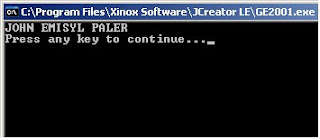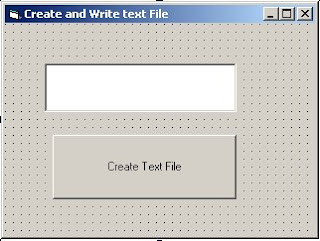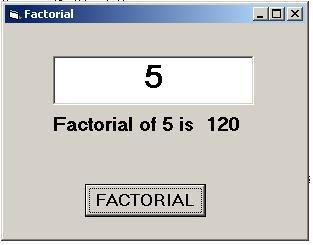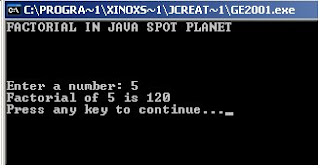Monday, January 26, 2009

How to display date and time using vb6.0?

Getting the time and Date in your computer basically its easy.
First is to put two labels in your form label1 and label2 that is the name of the two labels and then after that put timer in your form too.
Timer1 is the default name of the timer and set timer interval into 10. And double click your form which is your form1 and after that i want you to put this code inside your form load() . label1.caption is equal to Date and lablel2.caption is equal to Time after that run your program. You can download my example below.. Thats all!How to display date and time using vb6.0?

You may set first the TIME and DATE of your system i mean you computer because there are some cases that the time and date in your computer is not set so may set first before doing some actions inyour visual basic 6.0.
Getting the time and Date in your computer basically its very easy.
First is to put two labels in your form label1 and label2 that is the name of the two labels and then after that i want you put 1 command button inside you form which is command1. And double click your command button put this code inside command1_click() label1.caption is equal to Date and lablel2.caption is equal to Time after that run your program and try to click your command button and it will automatically appear the time and date. You can download my example below.. Thats all!How to use for each statement in Php?

I have here an example using foreach statement in Php the first thing you need to do
is to open notepad but if you have dream weaver or other software editor is OK.
Php has several conditional and loop statements that have been part of the
language since its creation. Unlike them, the foreach statement is one
of the loop statement influenced by other programming languages that
used foreach for years. For example java. Unlike the other conditional and loop
statements, the foreach statement is used only on arrays so basically foreach statement
accept only variable arrays. I have here an example that you can download using foreach statement.. You can post comments or question about other entities in Php or other languages.

How to use toLowerCase in java?

toLowerCase() is one the basic method of the string class.
I have here and example using toLowerCase() method converting the Upper case text into
lower case.

Example my name:

String name ="JOHN EMISYL PALER"; Declaration of variable.
To convert my name into lower case form heres the following syntax.

System.out.println(name.toLowerCase());How to use toUpperCase in java?

toUpperCase() is one also of the basic method of string class in java like toLowerCase() method.
toUpperCase() method is opposite of toLowerCase() method. toLowerCase() is converting Upper case text into Lower case. They have different action dealing with Strings or Text. I have here an Example for toUpperCase() method.

Example:

String name="john emisyl paler";

System.out.println(name.toUpperCase());How to display numbers using do while loop in php?

`do while statement is one of loop statement in Php thathas a specific condition depending the program flows basically do while loop and while loop are commonly the same but deffirent format of coding. The same with while loop that do whilestatement will automatically stop if the condition becomes false.`
Example:
int x=1;

do {

System.out.println("Number: " + x);
x++;

}while(x<=10);
`You can download my example below.Download File`

How to display numbers using while loop in php?

`while statement is one of loop statement in Php thathas a specific condition depending the program flows. The while loopstatement will automatically stop if the condition becomes false forexample if you creating a simple program displaying numbers thewhile loop statement will stop displaying numbers base in a given valueor the count of the loop on how many times the loop repeat.`

while (condition)
`code to be executed;`

Simple looping using while loop
`Example:\$i=1;while(\$i<=10)  {  echo "The number is " . \$i . "";  \$i++;  }Output:The number is 1The number is 2The number is 3The number is 4The number is 5Download File`

How to create text file in vb6.0?

Creating and Writing text in Text file or Notepad in Visual Basic 6.0 here the following procedure. In this example i have 1 text box in my form and 1 command button in my form. After clicking your command button basically it will create notepad and write text depending the text that you inputted in you text box. You can download this example below.How to use switch statement in Php?

`Very nice example for switch statement.`

If have many blocks and if you want to select one of you blocks actually you can use Switch statement.

The switch statement is used to shorting the code i mean the block of code instead using if..else..elseif.

`Syntax:The switch statementswitch (expression){case label1:  code to be executed if expression = label1;  break;  case label2:  code to be executed if expression = label2;  break;default:  code to be executedif expression is differentfrom both label1 and label2;}Example:switch (\$x){case 1:  echo "Index 1 ";  break;case 2:  echo "Index 2";  break;case 3:  echo "Index 3";  break;default:  echo "No number between 1 and 3";}If the value of variable \$x is 2 the output is Output:  Index 2`

\$names = array("Johnr","Mario","Merbert");

Example 2:
`\$names = "John";\$names = "Mario";\$names = "Merbert";`
`echo \$names . " and " . \$names . " are ". \$names . "'s neighbors";?>You can the element or the value of the array using there indeces or index.Example:echo \$name;Output:MarioDownload File`

How to decalre variable using Php

`Proper Format\$var_name = value;Example:`
`//Open parameter\$txt = "Hello World!";\$number = 25;\$echo(\$txt . "");\$echo(\$number);?> //Closing parameterOuput:Hello word25Download File`

How to create Factorial using vb6.0?Private Sub Command1_Click()

Dim x As Integer
Dim ctr As Integer
ctr = 1

For x = 1 To Val(Text1.Text)

ctr = ctr * x

Next x

Label1.Caption = "Factorial of " & Text1.Text & " is" & ctr

End Sub

How to declare array in vb6.0?

Example:

Dim myArrayName(0 To 5) As String

'The range of this array is 0 to 4 only.
'Data type is String it means you can store only string value in your array.

myArrayName(0)="John"
myArrayName(1)="Mario"
myArrayName(2)="Mark"
myArrayName(3)="Ailey"
myArrayName(4)="Merbert"

Sunday, January 25, 2009

How to create Factorial using java?

I have here an example getting the factorial of the given value in this example i used
import.java.io.*; its a kind of package in java in order the user will input a number.
IO means
Input and Output
. For example if the user will input 5 basically the factorial of 5 is 120 i have here a formula getting 120.

5 is the user input

solution
1 x 2 x 3 x 4 x 5 = 120

In order we can get the 120 in java we will use for loop statement on of the loop statement in java. Here the Output!

****
****OUTPUT**********

//I have here very nice example for do while.

class dowhile
{ //class name
public static void main(String[] args) { //default method of java
int x = 1;
do {
System.out.println("Number: " + x);
x++;
}while(x<=10);

/*
Instructions

x<=10 is your condition til the condition becomes false.
x++ is your Incrementation in-order your x will move starts 1 to 10.
System.out.println("Number: " + x); is for display Number : 1 to 10.

*/

}
}

********OUTPUT**********

How to use for loop in java programming?

//I have here very nice example for forloop.

class forloop
{ //Your class the name of the is 'forloop
public static void main(String[] args) {
for(int x=1; x<=10;x++) { //
System.out.println("Number: " + x);
}

/*
Instructions

int x=1 is your declaration of your variable starts from 1 to 10.
x<=10 is your condition til the condition becomes false.
x++ is your Incrementation in-order your x will move starts 1 to 10.
System.out.println("Number: " + x); is for display Number : 1 to 10

*/

}
}

/*
********OUTPUT**********

Number: 1
Number: 2
Number: 3
Number: 4
Number: 5
Number: 6
Number: 7
Number: 8
Number: 9
Number: 10